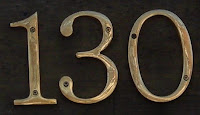## Thursday, April 28, 2011

### 130

130 = 2 x 5 x 13.

130 has two representations as a sum of two squares: 130 = 32 + 112 = 72 + 92.

130 is the smallest number with eight representations as a sum of four positive squares: 130 = 12 + 12 + 82 + 82 = 12 + 22 + 22 + 112 = 12 + 22 + 52 + 102 = 12 + 42 + 72 + 82 = 22 + 32 + 62 + 92 = 32 + 62 + 62 + 72 = 42 + 42 + 72 + 72 = 42 + 52 + 52 + 82.

130 is a cake number.

130 is the sum of the factorials of the first five terms of the Fibonacci sequence: 130 = 1! + 1! + 2! + 3! + 5!.

130 is the only number that is equal to the sum of the squares of its first four divisors: 130 = 12 + 22 + 52 + 102.The Lockheed C-130 Hercules is a four-engine turboprop military transport aircraft.